Probability Distribution

Chapter 13 Class 12 Probability
Serial order wise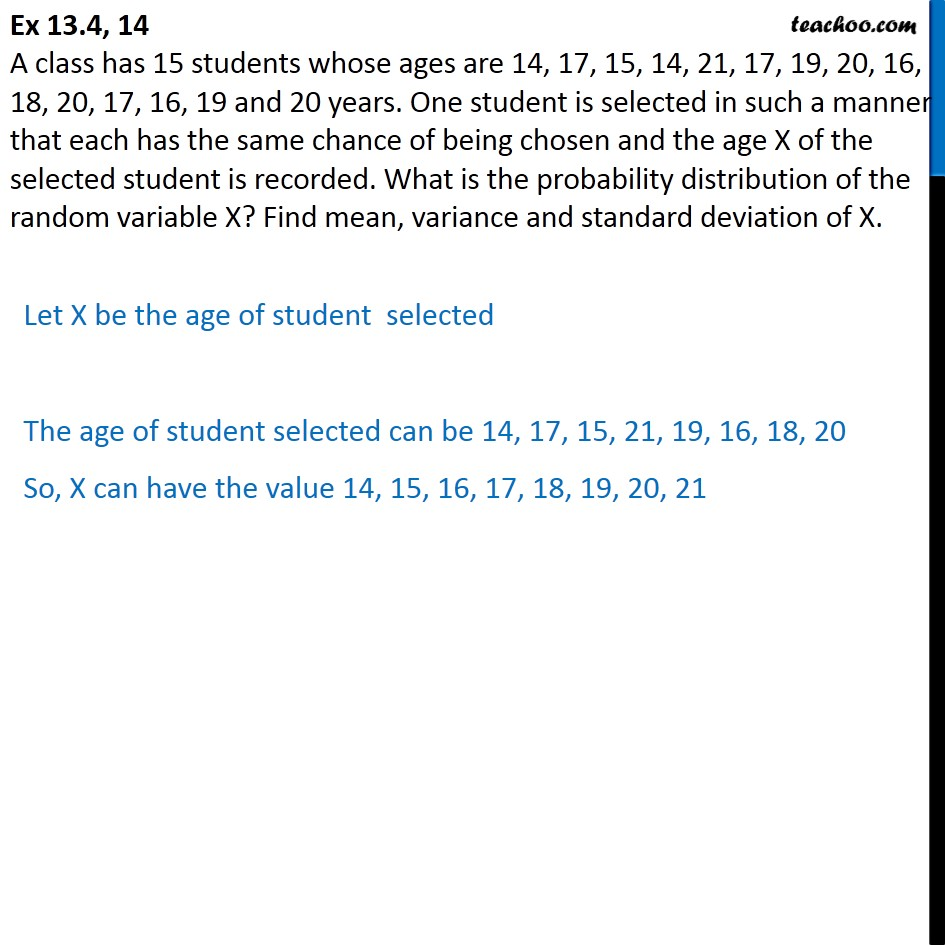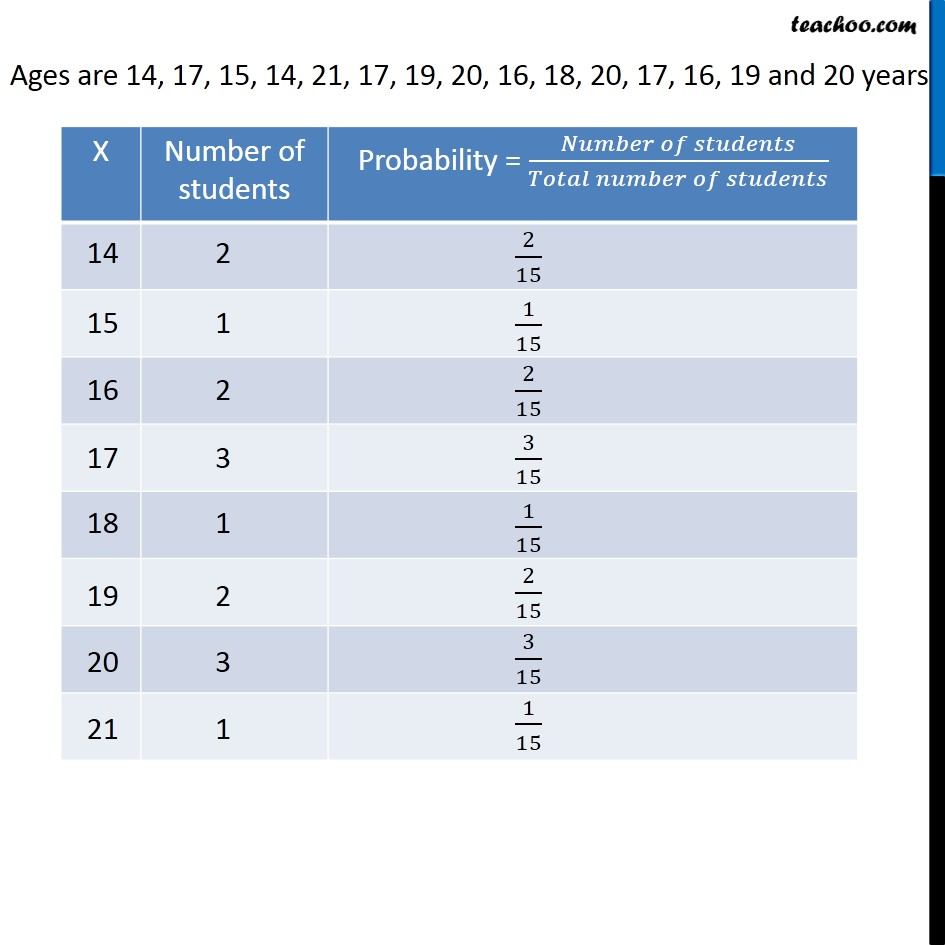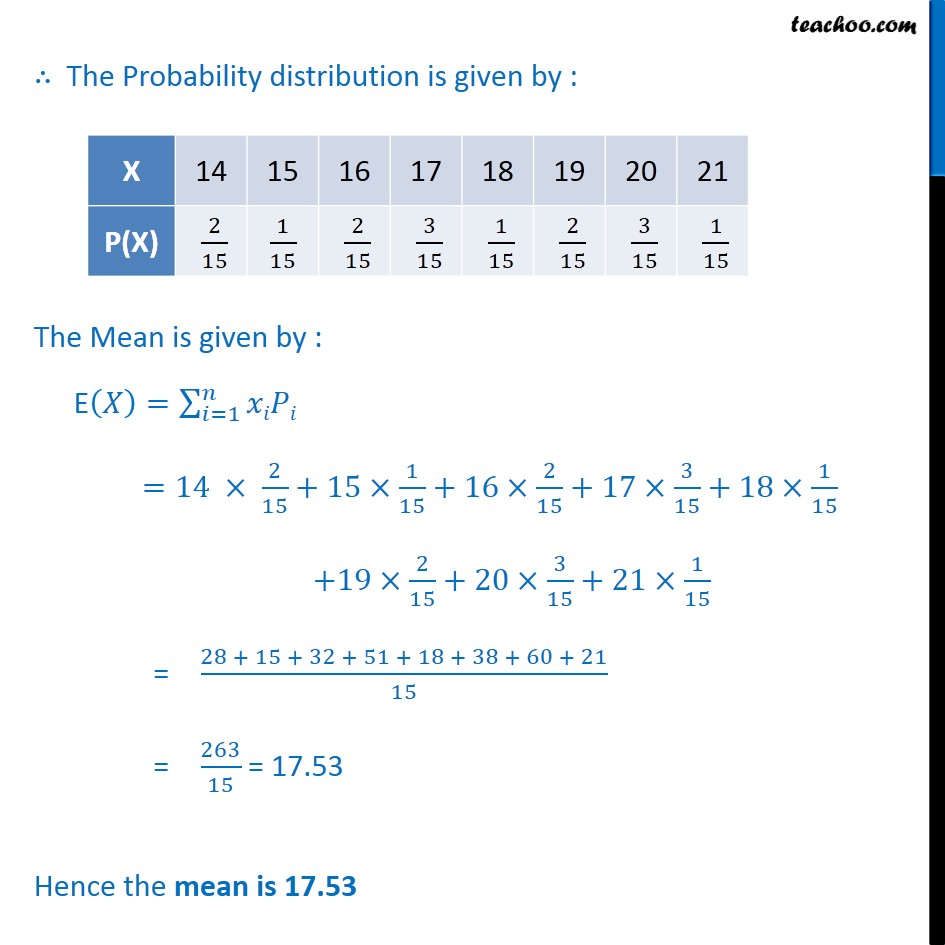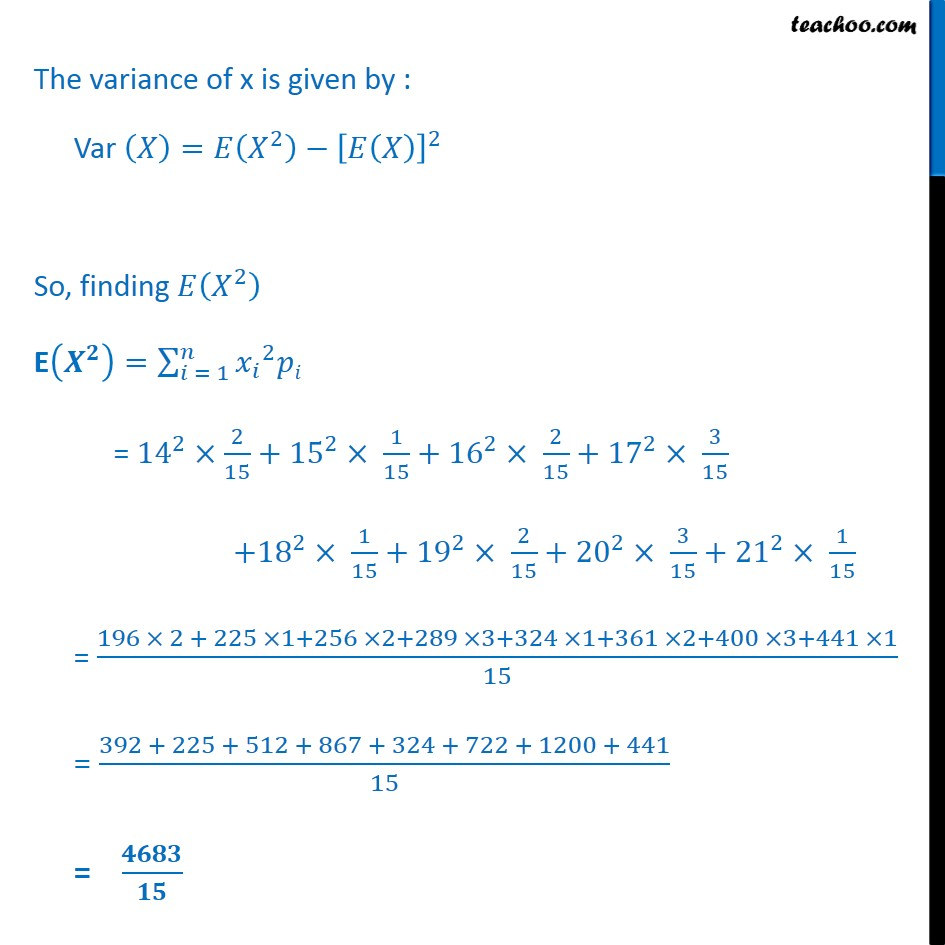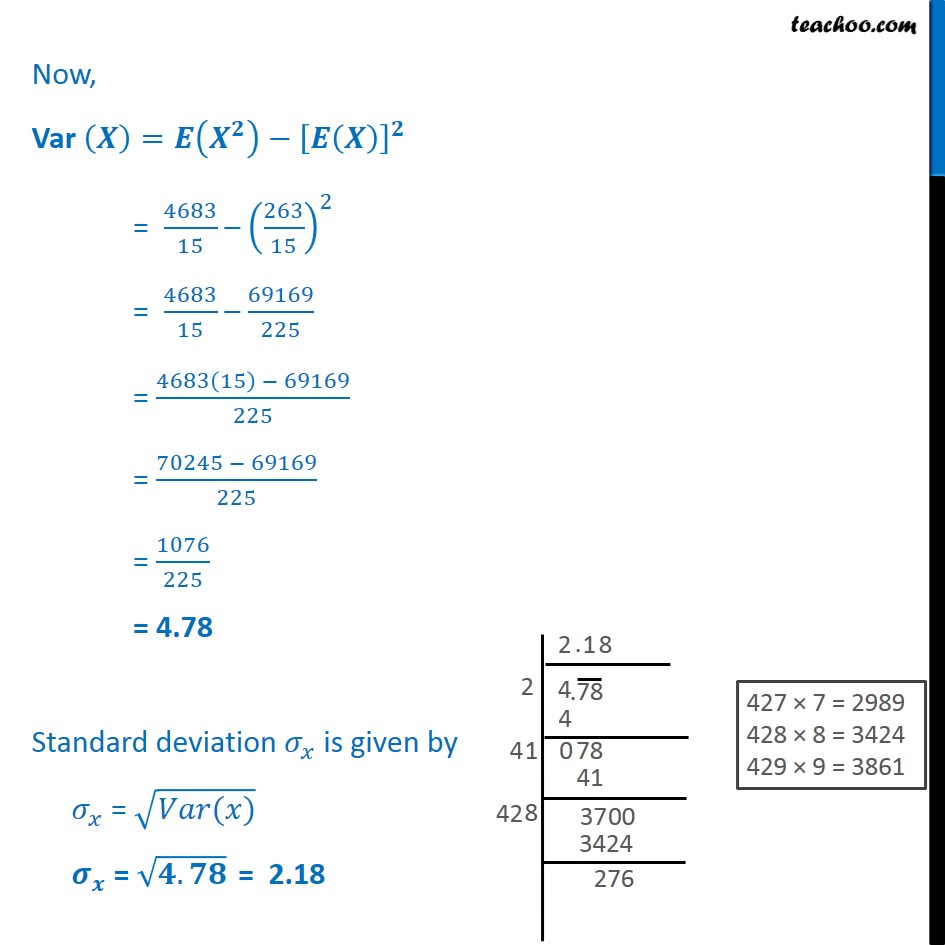Learn in your speed, with individual attention - Teachoo Maths 1-on-1 Class

### Transcript

Question 14 A class has 15 students whose ages are 14, 17, 15, 14, 21, 17, 19, 20, 16, 18, 20, 17, 16, 19 and 20 years. One student is selected in such a manner that each has the same chance of being chosen and the age X of the selected student is recorded. What is the probability distribution of the random variable X? Find mean, variance and standard deviation of X. Let X be the age of student selected The age of student selected can be 14, 17, 15, 21, 19, 16, 18, 20 So, X can have the value 14, 15, 16, 17, 18, 19, 20, 21 The Probability distribution is given by : The Mean is given by : E = =1 =14 2 15 +15 1 15 +16 2 15 +17 3 15 +18 1 15 +19 2 15 +20 3 15 +21 1 15 = 28 + 15 + 32 + 51 + 18 + 38 + 60 + 21 15 = 263 15 = 17.53 Hence the mean is 17.53 The variance of x is given by : Var = 2 2 So, finding 2 E = = 1 2 = 14 2 2 15 + 15 2 1 15 + 16 2 2 15 + 17 2 3 15 + 18 2 1 15 + 19 2 2 15 + 20 2 3 15 + 21 2 1 15 = 196 2 + 225 1+256 2+289 3+324 1+361 2+400 3+441 1 15 = 392 + 225 + 512 + 867 + 324 + 722 + 1200 + 441 15 = Now, Var = = 4683 15 263 15 2 = 4683 15 69169 225 = 4683 15 69169 225 = 70245 69169 225 = 1076 225 = 4.78 Standard deviation is given by = ( ) = . = 2.18